﻿ Use of Safety Light Curtains in Mechatronic Systems

### Use of Safety Light Curtains in Mechatronic Systems

Ján IvankaOPEN ACCESSPEER-REVIEWED

## Use of Safety Light Curtains in Mechatronic Systems

Department of Security Engineering, Tomas Bata University in Zlín, Czech Republic

### Abstract

The article describes some of the aspects of how the optosensors and laser scanners can be used in the field of security of protected areas and safety fields. The article presents some of the applied possibilities of measuring on spherical area using transmission formulas for gaining data and actual setting of active elements of the optosensors and laser scanners for the environment in a 3D application mechatronic system.

### At a glance: Figures

1
Prev Next

• Ivanka, Ján. "Use of Safety Light Curtains in Mechatronic Systems." American Journal of Mechanical Engineering 1.7 (2013): 194-197.
• Ivanka, J. (2013). Use of Safety Light Curtains in Mechatronic Systems. American Journal of Mechanical Engineering, 1(7), 194-197.
• Ivanka, Ján. "Use of Safety Light Curtains in Mechatronic Systems." American Journal of Mechanical Engineering 1, no. 7 (2013): 194-197.

 Import into BibTeX Import into EndNote Import into RefMan Import into RefWorks

### 1. Introduction

Up-to-date optical electric sensors are based on various physical methods, for instance triangulation, phase shift measurement, or pulse propagation techniques. The use of optical electric sensors, along with powerful digital processors, allows realisation of very efficient and economically effective measurements, sensing, and security tasks. Light beams as the instrument of measurement techniques are fascinating – they allow touch-less measurements in very fast sequences, their range is long and the resolution is still high; moreover, they are almost immune to any interference effects. Therefore, properties of any other measurement techniques are really very far from comparison with the range of applications using optical electric sensors. Any use of light for measuring distances, detection of objects and protection of persons is always based on reflection of a light beam from an object. For achieving excellent results, optical electric sensors use the method of triangulation combined with the Charged Couple Device (CCD) sensor technology. Optical electric sensors are equipped with a number of the high-resolution CCD sensors. Laser diodes are used as the light source for measuring in the range of 20 mm up to 250 m. Optical electric sensors allow measuring objects with the luminous intensity of 6% up to 90% in the entire measuring range and, thus, guarantee data almost independent of the luminous intensity. Measured values may be read in the analogue and digital form. The sensor itself can be comfortably "taught" either manually, or via a simple parameter setup through the RS-22, RS-485 or the Actuator/Sensor (AS) PC interfaces. Optical electric sensors based on the phase shift measurement provide absolute measured values in the range of 0.2 m up to 170 m. The resolution can be continuously regulated whereas the scatter in repeated measuring is ± 2 mm. These optical electric sensors read newly measured values every one millisecond and, thus, establish optimal conditions for functioning in fast position control loops. The red laser used significantly reduces requirements for installing, adjusting, and setting the sensor's position. Thanks to this flexibility of their properties, optical electric sensors are the ideal instrument for distance measuring in location detecting and positioning. In order to meet increasingly stringent requirements related to security of persons, it is imperative to ensure reliable security for the critical areas. Laser security scanners based on the pulse propagation can offer a number of genuine security benefits. The pulse propagation technique principle consists in spreading of light pulses emitted by a laser diode by means of a rotary mirror over the entire work area. This principle of measuring is suitable not only for security but also for a number of measurement applications. The scanner allows definition of up to four different couples of security areas. For process-dependent activities it is, then, possible to activate and deactivate each couple separately.

### 2. Security Light Curtains

The basic task of Safety Light Curtains (SLC) is to protect a person or other persons in the security zone area. Therefore, they must quickly detect intrusion of a person into the secured area and issue a respective signal about it. In the CSI, this primarily applies to securing of areas adjacent to buildings, outdoor garages, indoor garages, access roads and others. Security light curtains consist of separate transmitting and receiving units between which a protected area is created. The transmitting unit is equipped with a number of infrared (red) light sources transmitting cyclically short light pulses that normally strike respective light-sensitive sensors in the opposite receiving unit. However, when an opaque object enters the protected area and at least one beam is interrupted, i.e. the light pulse emitted does not strike the corresponding sensor, the receiving unit generates an output signal from which it is very easy to derive an alarm command. The width of the protected area is determined by the maximum range of the light curtain in which the sensor reliably receives all the light pulses transmitted; this range varies from zero up to several tens of metres. The height of the protected area is given by the design height of the transmitting and receiving units, which is normally the function of the number of transmitted light beams and the pitch between each other. The pitch, or the distance between neighbouring light beams, defines the resolution rate of the security light curtain and its effectiveness. The shorter the light beams pitch is, the smaller the object entering the protected area of the light curtain that can be detected. The resolution of the light curtain must correspond to the security level required. The evaluation electronics of security light curtains are either integrated into the receiving unit, or built in a separate casing that can be fixed on the wall. Nowadays, the electronics are usually based on a microprocessor controller or the customer Application-Specific Integrated Circuit (ASIC), which allows the easy addition of a number of useful functions widening the application range or enhancing the comfort of the light curtain operators. The LMS systems can also be used in the area of spatial scanning and detecting the size of vehicles, and the speed and direction of passing vehicles. Physically, the LMS laser scanner is based on spreading the laser beam in the infrared spectrum and calculating the response (return) time of the beam transmitted. The possible spreading angles are 360°, 180°, and 100° with the range up to 150 m. Basic parameters are assigned for correct setting of the active elements of LMS and PLS, and also constituents for doing the measuring on the spherical area.

### 3. Measuring on Spherical Area

Let us suppose that the receiver elements are moving within a spherical area (r, z) in the distance r = d from the rotation axis of the transmitter being measured. If we are to assume that the multiple rebounds between the two transceivers are small enough so that we can neglect them, we can thus deduce the transmission formula based on the basic Tauffer’s formulas with standard assumptions of linearity, constant frequency (ej) and the position in free space. It is not our aim to state here the deduction of needed relations, and because of that in the next part we will only give a very short statement of needed relations. [2, 3] Sense of polarization is used to determine whether ρrc(0)is higher or lower than the unit value (=1) as this information cannot be obtained from the ratio of A axes or angle of inclination τ. [1, 7] Considering selected definition of coordinates, main component for the single-output probe as well as for the Y port of the probe with two outputs will be the component E, having its component on the axis as follows: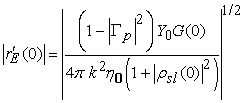(1)
Figure 1. Systems of coordinates used for calculation of measured values

In addition to rectangular 0xzy systems, definition of spherical systems firmly linked to the aerial is required in order to determine directions in space and vector components by means of two spherical angles. [3, 4] There is a wide array of options and we will choose three thereof. In mathematics, the most common system is that with z-axis as well as and angles, which is shown in Figure 1a. Figure 1b and Figure 1c show systems with A, E and α, ε angles. Respective direction in space can be specified using either propagation vector components k or whichever systém of angles, be it θ, φ; A,E or α, ε. Similarly, vector transverse to the radial direction of the sphere (direction of propagation) can be figured using three systems of spherical components θo, φo; Ao, Eo or αo, εo. [1, 2].

The transmission formula that determines the measured relative data of the safety field bo´(φ, z) (complex receiver outputs) in the terms of transmission parameters T(γ) for the receiver being measured, and actual transmission parameters of the appliance (γ), will be formally identical as the relation (2), only the individual variables will be defined in a different way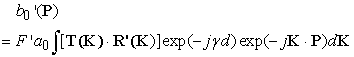(2)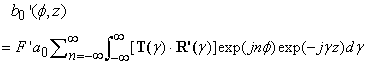(3)

where a0 is a complex input of the measured receiver, is determined by the mismatch between the receiver and transmitter, which we can express in this way: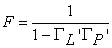(4)

ΓL´ and Γp´ are coefficients of the receiver and the transmitter. As the receiver does the scanning on the spherical area, the output is recorded bo´(φ, z) for 0≤ φ < 2π and –∞≤ z < ∞. This means that the basic parameters of the transmission media bo´(φ, z) get measured for all values φ and z. Practically the z is always limited to final scanning and it is assumed that the bo´(φ, z) is limited outside this area. The integral (2) can be changed with help of the Kirilian’s transformation to :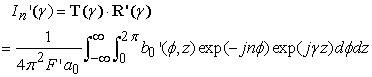(5)

Similarly as it is with scanning in level, even if we know the (γ) we cannot determine the T), since in (4) there occurs a scalar product. What we can do, however, is to carry out a second scanning with a different receiver. For the second independent scanner we can write analogically same relations as (3) to (5), only instead of bo´(φ, z) we will write bo´´(φ, z) and similarly for other quantities – this means we will use a0, F´´, ΓL´´, Γp´´, R´´) and In´´(γ). Formula (1) and formula for In´´(γ) can be written in these constituents: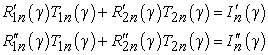(6)

Solving these relations (6) we will get: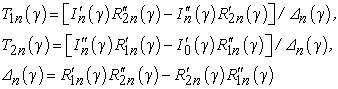(7)

The determination of the reception coefficients of the receiver ) can be done based on the known emitting characteristics of the sensing probe (which we can determine either by measuring or by calculation). If we know the reception functions of the sensing probe R) with cylindrical system that has its central point on the probe, we can get the ) by means of: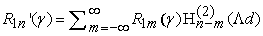(8)

where Hm(2)(x) is Hankel’s function of the second kind, of the m order, Λ=(k2-γ2)1/2, d is the distance of the probe from the rotation axis of the measured antenna. We can write a similar formula for R1n´´(γ). This formula expresses a very interesting and useful property. Even if the reception function of the probe R) can have a negligibly small number of members, it will give the R1n´(γ) for many values n. This means that the (8) can be used even with small receivers which are described by several spherical modes m, since the number of modes is determined similarly as for the measured spherical area. It is obvious that for the calculation of emitting characteristics we only have to program the relations (5) and (7), where we get the In´(γ) from the measured values bo´(φ, z) with help of the relation (3) and similarly we will get the In´´(γ) from the measured values bo´´(φ, z). To do the calculations, we will need the following measured quantities: d is the distance between the receiver and the rotation axis of the measured appliance; ΓL´ and Γp´ are the coefficients of the receiver;bo´(φ, z)/ ao the size and the relative phase of the transmission between the input of the measuring of the transmitter, and the output of the receiver. Usually the measuring bo´(φ, z) will be normalized in two steps given by analogical expression, such as: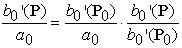(9)

where P0 is a selected fixed point. That means that in the beginning we will select a fixed point, e.g. the beginning of coordinates P0 =(0,0), where we will normalize the outcome of the bore, and then we will normalize all other measurements to this point. Using the FFT for the calculation of the relation (9) – and analogically for (4) – problems may occur due to the fact that the number of samples does not respond with power 2, which is required by algorithms (when using the MATLAB program it would be possible to use any number of samples, but the used algorithm is significantly slower than the algorithm for N=2M). In this case it is possible to complement the unmeasured values with nulls (for great values r and s which are located “in the middle” of the entry vector), and when doing the measuring in line (or in level) the algorithms do not change. A little more complex situation will come up when scanning in the angle of φ. Then for the addition of Δφ 1/2a radians which is not dependent on the distance of the receiver from the measured transmitter, we will gain the space of the same size k1= 2π1 regardless of the number of samples (inclusive of “stuffing” with nulls). We assume for the simplification of the sum in the relation (5) – for R of the samples – in this manner: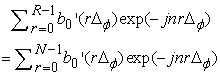(10)

since the b0´(rΔφ) are zero for r R. Let Δφ = 2π/R (usually the R shall be selected to be an even number). In order for us to use the relation: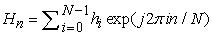(11)

for the given Δφ the n must be changed to n´=nR/N. Then, we will get jn´rΔφ =j2πnr/N. That means that with filling, only the “measure” n will change. Transformations are usually required if the measurement in one system is prescribed (e.g. θ,φ) while another system is to be used for final results (e.g. A,E).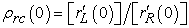(12)

### 4. Conclusion

At present times, the possibilities of using the laser and optoelectronic sensors in the field of securing mechatronic constellations and systems are stunning. This involves securing spaces of horizontal and vertical orientation of the safety field, or securing spaces in 3D applications, as well as monitoring the entrances into objects, guarding flat roofs, guarding side facades with a possibility to set up alarm zones with resistance to weather changes, or applications via float functions.

### References

  Forcek,V.: Numerical simulations of problems occurring in near-field and measurements. 7th Colloquium on Laser Communication, Belgium, 2004, pp. 422-481.In article  Yaghjian, A. D.: Near-field measurements on a cylindrical surface: A source scattering-matrix formulation. National Bureau of Standards Technical Note 696. Boulder, 45-58, Sep. 2006.In article  Newell, A. C. - Lee, D.: Application of the NIST 18 term errormodel to cylindrical near-filed field measurements. Antenna Measurement Techniques Association Conference, Oct. 2000.In article  Repjar, A. G. - Newell, A. C. - Francis, M. H.: Accurate determination of planar near-field correction parameters for linearly polarized probes. IEEE Trans. Antennas Prop., AP-36, N. 6, pp. 855-768.In article  Press, W. H. a col.: Numerical recipes. Cambridge, Cambridge University Press 1992.In article  Frankovský, Peter., Pástor, Miroslav., Kenderová, Mária.: Automatization of Strain and Stress Fields Assessment Based on the Principles of Reflection Photoelasticity. In: EAN 2012 : Proceedings of the 50th annual conference on experimental stress analysis. - Praha : Czech Technical University of Prague, 2012.In article  Valouch, Jan. Electromagnetic Compatibility of Alarm Systems - Legislative and Technical Requirements. In: Security Magazin. Issue No 106, 2/2012. Praha: Security Media, 2012. p. 32-36.In article